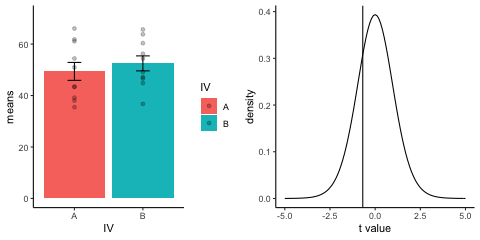{{ message }}

# Animation Composition

Thomas Lin Pedersen edited this page Aug 17, 2018 · 1 revision

# Placing Animations side-by-side with magick

submitted by Matt Crump

In the example I simulate sample scores and means for an independent sample t-test. In one panel I plot the means and scores, in the other panel I show the t-distribution and the observed t for each simulation.

In order to show them at the same time the animations are then combined using `magick`

``````library(dplyr)
library(ggplot2)
library(magick)
library(gganimate)

A<-rnorm(100,50,10)
B<-rnorm(100,50,10)
DV <- c(A,B)
IV <- rep(c("A","B"),each=100)
sims <- rep(rep(1:10,each=10),2)
df<-data.frame(sims,IV,DV)

means_df <- df %>%
group_by(sims,IV) %>%
summarize(means=mean(DV),
sem = sd(DV)/sqrt(length(DV)))

stats_df <- df %>%
group_by(sims) %>%
summarize(ts = t.test(DV~IV,var.equal=TRUE)\$statistic)

a <- ggplot(means_df, aes(x = IV,y = means, fill = IV)) +
geom_bar(stat = "identity") +
geom_point(aes(x = IV, y = DV), data = df, alpha = .25) +
geom_errorbar(aes(ymin = means - sem, ymax = means + sem), width = .2) +
theme_classic() +
transition_states(
states = sims,
transition_length = 2,
state_length = 1
) +
exit_shrink() +
ease_aes('sine-in-out')

a_gif <- animate(a, width = 240, height = 240)

b <- ggplot(stats_df, aes(x = ts))+
geom_vline(aes(xintercept = ts, frame = sims))+
geom_line(aes(x=x,y=y),
data = data.frame(x = seq(-5,5, .1),
y = dt(seq(-5,5, .1), df = 18))) +
theme_classic() +
ylab("density") +
xlab("t value") +
transition_states(
states = sims,
transition_length = 2,
state_length = 1
) +
exit_shrink() +
ease_aes('sine-in-out')

b_gif <- animate(b, width = 240, height = 240)